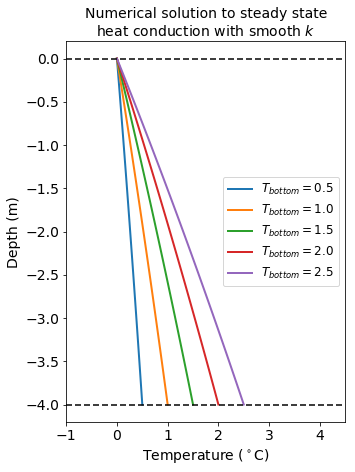# 1D heat conduction (layered medium)#

Geodynamics

## 1D steady state heat equation#

Consider a 1D model with two layers of different thermal conductivities - $$k_U$$ for layer $$0 < y < a$$ and $$k_L$$ for layer $$a < y < b$$. In either layer, no heat is generated.

Boundary conditions:

• temperature at $$y=0$$ is $$T_s$$

• temperature at $$y=b$$ is $$T_m$$

The governing ODE for each layer is:

$k\frac{d^2T}{dy^2}=0.$

### Analytical solution#

Analytically, the ODE can be solved separately for upper and lower layer.

Upper layer ODE can be written as

$k_U\frac{d^2 T_U}{dy^2}=0.$

Integrating ODE once with respect to $$y$$ we obtain

$\frac{dT_U}{dy}=A,$

where $$A$$ is a constant of integration. Integrating once again, we get

$T_U(y) = Ay+B.$

Now, using boundary condition at $$y=0$$:

$T_U(0)=A\times 0 + B =T_s\Rightarrow B = T_s,$
$T_U(y)=Ay+T_s.$

Similarly for lower layer ODE is

$k_L\frac{d^2 T_L}{dy^2}=0 \Rightarrow T_L(y)=Cy+D.$

Using boundary condition at $$y=b$$, we get:

$T_L(b)=Cb+D=T_m\Rightarrow D=T_m-Cb,$
$T_L(y)=T_m+C(y-b).$

We have two unknown constants $$A$$ and $$C$$. For that, we need two more boundary conditions:

• temperature is continuous at the interface:

$T_L(a)=T_U(a)$
• heat flux is continuous at the interface

The first condition yields:

$Aa+T_s=T_m+C(a-b),$
$A=\frac{T_m-T_s+C(a-b)}{a}.$

The solutions for both layers are then:

$T_U =T_s+[T_m-T_s+C(a-b)]\frac{y}{a},$
$T_L = T_m+C(y-b).$

The upwards heat flux into the interface from the lower layer is:

$k_L\frac{dT_U(y)}{dy}\Big|_{y=a}=k_LC.$

The upwards heat flux away from the interface into the upper layer is:

$k_U\frac{dT_L(y)}{dy}\Big|_{y=a}=k_U[T_m-T_s+C(a-b)].$

Equating these two heat fluxes we get:

$k_LC=k_U[T_m-T_s+C(a-b)],$
$C=\frac{k_U(T_m-T_s)}{ak_L+(b-a)k_U}.$

Therefore, the analytical solution yields:

$T_U = T_s + (T_m-T_s) \Big[\frac{k_L}{ak_L +(b-a)k_U}\Big] y,$
$T_L = T_m + (T_m-T_s) \Big[\frac{k_U}{ak_L +(b-a)k_U}\Big] (y-b).$

We can calculate and plot the analytical solution with the following code:

import matplotlib.pyplot as plt
import numpy as np

# Set boundary temperature
T_s = 0
T_m = 100

# Set y
N = 51
L = 30
y = np.linspace(0,L,N)

# Set thermal conductivities
k_upper = 1
k_lower = 2

# Set layer thickness
a = y[int(N/2)]
b = y[-1]

# Calculate tempearture profile
T = np.zeros(N)

for i in range(len(T)):
if y[i] <= a:
T[i] = T_s+(T_m-T_s)*(k_lower/(a*k_lower+(b-a)*k_upper))*y[i]
else:
T[i] = T_m +(T_m-T_s)*(k_upper/(a*k_lower+(b-a)*k_upper))*(y[i]-b)

plt.figure(figsize=(5,7))

plt.plot(T, -y, color="forestgreen", lw=2)

plt.axhline(0, color="black", dashes=(4,2))
plt.axhline(-y[int(N/2)], color="black", dashes=(4,2))
plt.axhline(-y[-1], color="black", dashes=(4,2))

plt.xlabel("Temperature", fontsize=14)

plt.yticks([0, -y[int(N/2)], -y[-1]], ["$y=0$", "$y=a$", "$y=b$"], fontsize=14)
plt.xticks(fontsize=14)

plt.text(T[-1]*3/4., -y[int(N/4)], "$k=k_U$", fontsize=14)
plt.text(T+5, -y[int(3*N/4)], "$k=k_L$", fontsize=14)
plt.text(T, -y+1, "$T=T_s$", fontsize=14)
plt.text(T[-1], -y[-1]-2, "$T=T_m$", fontsize=14, ha='right')

plt.ylim(-y[-1]-4, -y+4)
plt.xlim(T-5, T[-1]+5)

plt.title("Analytical solution to steady state\nheat equation with 2 layers",
fontsize=14)

plt.show()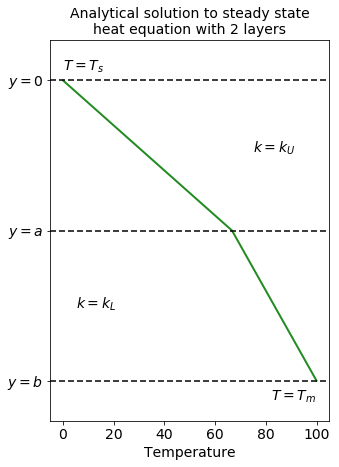### Numerical solution#

In the previous section, we showed how to analytically solve 1D steady state heat equation for two layers with different thermal conductivities. Next, we will create a numerical solution.

#### Discretisation#

The steady state ODE for heat equation in one dimension for material with uniform thermal conductivity states:

$k\frac{d^2T}{dy^2}=0.$

However, since our model has two layers of different conductivities, the equation becomes instead:

$\frac{\partial}{\partial y}\Big(k\frac{\partial T}{\partial x}\Big)=0.$

Using chain rule, we obtain:

$\frac{\partial k}{\partial y}\frac{\partial T}{\partial y}+k\frac{\partial^2T}{\partial y^2} =0.$

To discretise the equation, we will use finite difference methods. First derivative of temperature and thermal conductivity are described as

$\left(\frac{\partial T}{\partial y} \right)\bigg\rvert_{y=y_i} \approx\frac{T_{i+1}-T_{i-1}}{2\Delta y}, \quad \left(\frac{\partial k}{\partial y}\right) \bigg\rvert_{y=y_i} \approx\frac{k_{i+1}-k_{i-1}}{2\Delta y}.$

The second derivative of temperature is

$\left(\frac{\partial^2 T}{\partial y^2}\right)\bigg\rvert_{y=y_i}\approx\frac{T_{i+1}-2T_{i}+T_{i-1}}{(\Delta y)^2}.$

Now, we can substitude these into our equation:

$\frac{k_{i+1}-k_{i-1}}{2\Delta y}\frac{T_{i+1}-T_{i-1}}{2\Delta y}+k_i\frac{T_{i+1}-2T_{i}+T_{i-1}}{(\Delta y)^2}=0\quad ||\cdot 4(\Delta y)^2,$
$(k_{i+1}-k_{i-1})(T_{i+1}-T_{i-1})+4k_i(T_{i+1}-2T_i+T_{i-1})=0,$
$8k_i T_i = (k_{i+1}-k_{i-1})(T_{i+1}-T_{i-1}) +4k_i(T_{i+1}+T_{i-1}).$

And finally, we obtain the solution for temperature at $$y_i$$:

$T_i = \frac{(k_{i+1}-k_{i-1})}{8k_i}(T_{i+1}-T_{i-1}) +\frac{1}{2}(T_{i+1}+T_{i-1}).$

Code below shows the implementation and plot of obtained temperature profile:

# Set temperature arrays
T_old = np.zeros(N)
T_new = np.zeros(N)

# Set boundary conditions
T_old = T_s
T_old[-1] = T_m

# Create y array
y = np.linspace(0,L,N)

# Create k array and
# substitute correct conductivities
k = np.zeros(N)

for i in range(len(k)):
if i <= int(N/2):
k[i] = k_upper
else:
k[i] = k_lower


To obtain the temperature profile, we need to update $$T_{old}$$ multiple times to make sure our steady state solution converged. We will use RMS error to see how our solution converges as compared to the analytical solution:

from sklearn.metrics import mean_squared_error

nf = 1000

rms = np.zeros(nf)

for it in range(nf):

T_new = T_old

for i in range(1,N-1):
T_new[i] = (k[i+1]-k[i-1])/(8*k[i])*(T_old[i+1]-T_old[i-1])+1./2.*(T_old[i+1]+T_old[i-1])

rms[it] = np.sqrt(mean_squared_error(T, T_new))

plt.figure(figsize=(7,5))
plt.plot(rms, color="magenta")
plt.xticks(fontsize=12)
plt.yticks(fontsize=12)
plt.xlabel("Iteration", fontsize=12)
plt.ylabel("RMS Error", fontsize=12)
plt.show()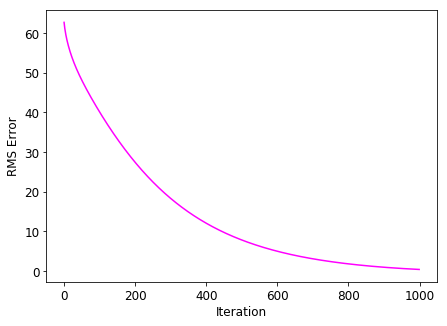Now we can plot the numerical solution:

plt.figure(figsize=(5,7))

plt.plot(T_new, -y, color="blue", lw=2)

plt.axhline(0, color="black", dashes=(4,2))
plt.axhline(-y[int(N/2)], color="black", dashes=(4,2))
plt.axhline(-y[-1], color="black", dashes=(4,2))

plt.xlabel("Temperature", fontsize=14)

plt.yticks([0, -y[int(N/2)], -y[-1]], ["$y=0$", "$y=a$", "$y=b$"], fontsize=14)
plt.xticks(fontsize=14)

plt.text(T_old[-1]*3/4., -y[int(N/4)], "$k=k_U$", fontsize=14)
plt.text(T_old+5, -y[int(3*N/4)], "$k=k_L$", fontsize=14)
plt.text(T_old, -y+1, "$T=T_s$", fontsize=14)
plt.text(T_old[-1], -y[-1]-2, "$T=T_m$", fontsize=14, ha='right')

plt.ylim(-y[-1]-4, -y+4)
plt.xlim(T_old-5, T_old[-1]+5)

plt.title("Numerical solution to steady state\nheat equation with 2 layers",
fontsize=14)

plt.show()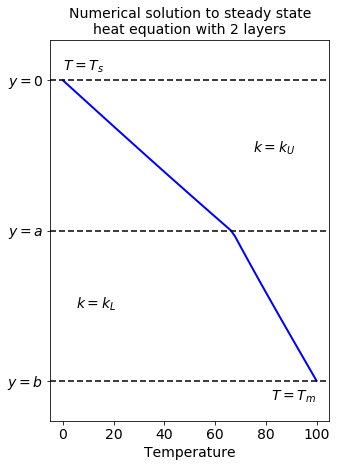### Numerical vs. analytical solution#

To compare the solutions, we will plot them and their difference at the same graph:

fig, axes = plt.subplots(1, 2, figsize=(8,6),
sharey=True)

ax1 = axes
ax2 = axes

ax1.plot(T, -y, color="forestgreen", dashes=(4,2),
lw=3, label="Analytical solution", zorder=2)

ax1.plot(T_new, -y, color="blue", dashes=(6,2),
lw=3, label="Numerical solution", zorder=1)

ax1.legend(loc="best", fontsize=12)

ax1.set_xlabel("Temperature", fontsize=12)
ax1.set_ylabel("Depth", fontsize=12)

ax2.plot(T_new-T, -y, color="magenta",
lw=2, label="$T_{num}-T_{analytical}$")

ax2.set_xlabel("Residual temperature",
fontsize=12)

ax2.legend(loc="best", fontsize=12)

# Create root mean scquared error
rms = np.sqrt(mean_squared_error(T, T_new))

ax2.set_title("RMS Error = %.4f" % rms)

plt.show()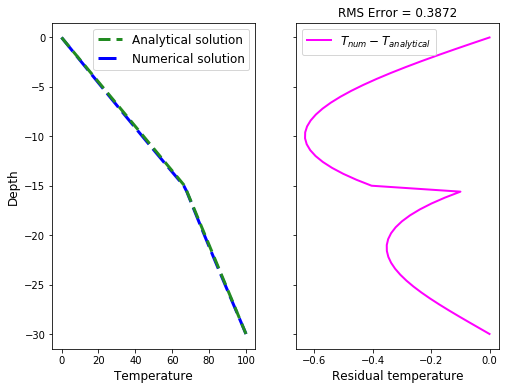As we can see on the graphs, the numerical solution is very close to the analytical solution.

### Three layers#

The same numerical analysis can be applied to multiple layers with different thermal conductivites. Here we show an example of three layer model: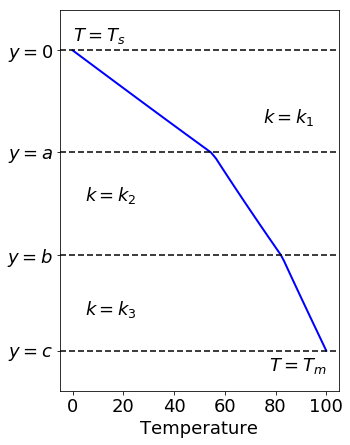### Smooth k#

So far, we created a piece wise function of thermal conductivity. In real life, it is more often the case that $$k$$ varies smoothly in space, especially at shallow depths.

Fisher et al. (2001) provides a relationship between depth and thermal conductivity for top $$4\,m$$ in the Alarcon basin based on field survey:

$k = 0.70 + 0.0035\times \text{depth}.$

According, to Figure 2 from the paper, we could take top temperature at $$0^\circ C$$ and bottom temperature between $$0.5^\circ C$$ and $$2.5^\circ C$$.

The numerical solution for these temperatures then looks: